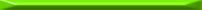Tags SICP

The code for this section is in Scheme.

So, we commence with chapter 3 of the book – Modularity, Objects, and State. The video lecture relevant to this section – 5a was, in my opinion, excellent. The way Sussman modeled assignment as a point in time was very lucid. He also explained the environment model of evaluation quickly and efficiently. Even when all these concepts are long familiar to you, learning a new way to look at things helps a lot. The approach taken in SICP to fully understand how the language works under the hood is very educational.

#### Exercise 3.1

This is very similar to the bank account example, except for a small twist – we want to be able to provide an initial value for the accumulation. Since this value is accepted by `make-accumulator`, the correct way to place it is in this function’s argument list.

```(define (make-accumulator initial)
(let ((accumulator initial))
accumulator)))
```

Note that we don’t need `begin` here, because the body of a `lambda` definition is implicitly wrapped in one.

#### Exercise 3.2

We’ll use the `dispatch` method employed in the last `make-account` example:

```(define (make-monitored proc)
(let ((call-count 0))
(define (dispatch m)
(cond
((eq? m 'how-many-calls?) call-count)
((eq? m 'reset-count) (set! call-count 0))
(else
(set! call-count (+ 1 call-count))
(proc m))))
dispatch))
```

#### Exercise 3.3

The modification is only to `dispatch` – the rest of the code should know nothing about passwords:

```(define (make-account balance password)
(define (withdraw amount)
(if (>= balance amount)
(begin (set! balance (- balance amount))
balance)
"Insufficient funds"))
(define (deposit amount)
(set! balance (+ balance amount))
balance)
(define (dispatch pass m)
(cond ((eq? m 'withdraw) withdraw)
((eq? m 'deposit) deposit)
(else (error "Unknown request -- MAKE-ACCOUNT" m)))
dispatch)
```

A part of this function may not be obvious, and although the book explains it I want to dwell on this point a little.

The local bindings created by `define`’s arguments aren’t different from using the `let` form1. So, `password` and `balance` are captured inside the definition of `make-account` the same way `call-count` of exercise 3.2 is. When the interpreter executes the definition of `make-account`, it also executes the definition of `dispatch`, with an environment that contains `balance` and `password` having the values passed to `make-account`.

#### Exercise 3.4

```(define (make-account balance password)
(define (withdraw amount)
(if (>= balance amount)
(begin (set! balance (- balance amount))
balance)
"Insufficient funds"))
(define (deposit amount)
(set! balance (+ balance amount))
balance)
(define (dispatch pass m)
(begin
(cond ((eq? m 'withdraw) withdraw)
((eq? m 'deposit) deposit)
(else (error "Unknown request -- MAKE-ACCOUNT" m))))
(begin
The weird combination of `printf` and `(lambda (x) x)` in the end of `dispatch` allows me to signal an error and yet not trigger an exit of the interpreter on first error. It would exit otherwise, since `dispatch` must return a function in order to work properly.1 In fact, `let` can be implemented using `lambda`.